# Statistics in the Triad, Part VIIIa: Smoothing, or Where the Data Are not Concentrated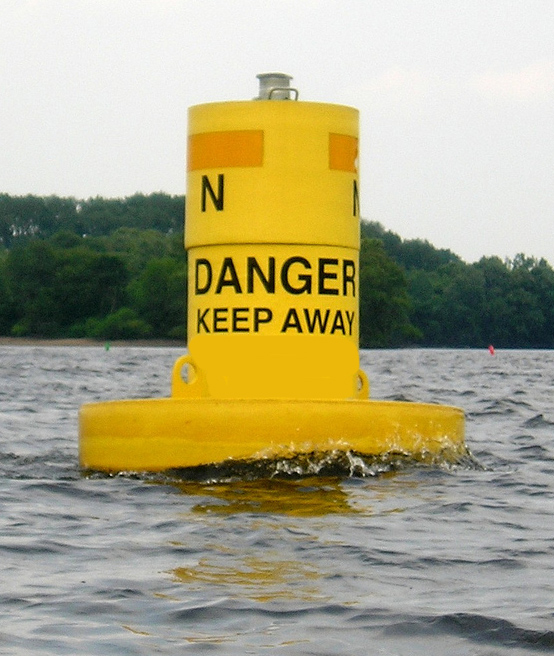This post is a cautionary tale — a warning buoy, if you will — about a widely-used method to aggregate and smooth data. The caution applies only to SenseMaker projects, which use ternary plots (triads) for both data collection and display, in particular those projects which yield high concentrations of data points near vertices. For now, we encourage SenseMaker practitioners to steer clear, though we expect to find a way to address the problem described below and will revise the post accordingly. All other KDE/PDF users full speed ahead.

### Data distribution in a triad

In a normative SenseMaker triad, story data are clustered at the three vertices plus the centroid of the triangle, with lesser groups near the midpoints of the sides and perhaps “stringers” between the centroid and the other six loci. The first image in Part III: Random Data shows an example.

There are two major reasons to examine the distribution of the data more closely. Firstly, if there are a lot of data points in one location, the true density of the data may be obscured due to overprinting. Secondly, comparison between groups of respondents is universal in SenseMaker projects, which generally leads to questions best answered with quantitative methods. Here are some possible comparative approaches:

• side-by-side visual inspection of cohorts or demographic groups, in the same or different triads, utilizing the client’s knowledge of the subject population(s);
• calculation of the statistical significance of the difference between the (geometric) means of two or more data groups, as illustrated in Part IV: Confidence Regions;
• selective estimation of data distribution by counting points in prominent clusters (see below); and
• histogram-like methods for aggregating data, either with discrete (discontinuous) bins or with smooth (continuous) contour lines. The first of these produces a ternary heat map, which is discussed in Part VIIIb. The second is something else entirely and the subject of this post.

This figure shows a triad, T7 (left-hand frame), from a recent project. Laurie chose the circled clusters by eye and used the “lasso” tool in Tableau to measure the number of points in each cluster. The labels show that 87% of the total falls in those seven clusters, with a slight overall majority in the elongated field at the upper vertex. This semi-quantitative method can be useful for a client’s discussions, for example, about differences between respondents in each cluster. If the number of stories increases substantially, however, then distinct clusters may become less obvious; and overprinting (evident near the top vertex where individual blue dots blur into an unresolvable blob) may become a barrier to seeing both the deconstructed detail and the larger structure.

### Aggregating and smoothing

The other two frames of the T7 figure are designed to show precisely that larger structure. The blue lines (center) and orange-shaded areas (right) mark contour lines that enclose areas of the triad with successively higher likelihood of containing story points. Hence, the most closely-packed blue lines and deepest-orange colors are near the upper vertex where the 54%-cluster of points was demarcated in the left frame. T7 is typical of the kind of match one would expect between contour patterns and the underlying data.

Just as on a topographic map, where contours indicate increasing height above sea level, the contours on these two plots can be read as a central ridge, climbing toward a peak at the upper vertex. These contours, however, trace out values of a probability density function (PDF), which were calculated by kernel density estimation (KDE). If you recognize the PDF and KDE acronyms, then you probably don’t need to go to those two Wikipedia links; and if you don’t, well, then you probably don’t want to go to them. (But, if you just can’t resist the lure, then go to the KDE link and read the first section on “Definition” and look at the side-by-side images comparing a histogram with its equivalent one-dimensional KDE. Now all you have to do is imagine that the T7 frames (above) are a two-dimensional version of the same idea. That histogram + KDE image is also reproduced in the Appendix at the end of this post.)

Suffice to say that the KDE + PDF combo is a way to aggregate the triad data and then calculate a smooth, continuous guess (the contours) about where any unsampled stories would be likely to plot. Again, T7 is a good example of the kind of match this technique can yield. The beauty of it is that the guess is non-parametric, meaning you don’t have to know anything about whether the data obey some particular set of statistical rules, such as a normal, bell-shaped distribution.

### When the data and contours don’t match

So the idea in principle is that the KDE contours will show the larger, overall structure of the data, whether there is overprinting or not. In some situations it would not be unusual to omit the data entirely, for example, where there were so many points that the image at some working scale was a blur of run-together dots. Fortunately, in the study of which T7 was a part, Laurie kept the data points as a base layer for the two versions of the KDE plots. That made it much easier to see the problem in T3:

In a nutshell, the contour pattern is wrong. Most importantly, there should be indication in the contours that the highest concentration of data is at the lower-left vertex, with equal secondary concentrations at the lower-right vertex and at the centroid. Instead, there is a single central peak, with all contours dropping off to the corners. If you were looking at this pattern on a topographic map while out hiking, you would expect to look up and see a familiar Alpine glaciation feature. Think “Matterhorn.” The actual data, however, describe a more subdued version of the KDE plots for T7, but rotated so that the “ridge” would be climbing up toward the lower-left vertex, with similar lower levels (probabilities) at the lower-right and center .

In fact, this is not an isolated example. In the study from which T3 and T7 were taken, these two are the extremes, and the other five triads can be arranged gradationally between them. Four of the seven, including T3, have contours that overall do not accord with the data. (Laurie has learned subsequently of at least three other SenseMaker practitioners who have seen this issue.)

### What might be going on

The most likely potential explanation gets into some very deep technical weeds, involving the interplay between the effect of the log-ratio transformation (LRT) on near-vertex and other near-zero triad data and the behavior of a fixed-width kernel function when data points are sparse. Oddly enough, it appears that the LRT preferentially stretches-out near-vertex/near-zero data so that they are less heavily weighted in the KDE than they should be. There is a long Appendix (below) that discusses these issues with some arithmetic detail, but very little mathematics, for the tiny number of people in the Cogniverse who might be interested.

Right now, we can say three things with a modest degree of certainty:

• Laurie’s data prep and Nicholas Hamilton’s KDE-related R code (see ggtern) were identical for all seven triads, so (simple) human error is unlikely to be the culprit. Said differently, non-SenseMaker practitioners should continue to have full confidence in ggtern.
• The ternary heat maps that are now available in ggtern are a reliable way to present the same information in a discrete format, though they admittedly lack the appeal of the continuous PDF contours. See Part VIIIb in this series for examples.
• Any SenseMaker practitioners who decide (or continue) to use KDE/PDF contours should include story data points as a base layer and heed the paraphrased lesson of Part VII: Mapping the Datasaurus Dozen: Don’t just calculate, plot… and then compare!

## Appendix

### The lowly histogram

The simplest way to grasp the likely cause of the mismatch between triad story data and PDF contours, as in the T3 example (above), is to look at the equivalent phenomenon in one dimension (1-D), that is, in a histogram and its corresponding KDE. As a reminder, even though a triad has three components and coordinates — (A,B,C), one at each vertex, and (a,b,c), each running from 0 to 1, respectively — only two are independent due to the closure constraint. Hence, the triad is a 2-D construct.

Here is a superb illustration  from the KDE article in Wikipedia:Comparison of the histogram (left) and kernel density estimate (right) constructed using the same data. The 6 individual kernels are the red dashed curves, the kernel density estimate the blue curves. The data points are the rug plot on the horizontal axis.

And here is the accompanying description of this graphical comparison (emphasis and footnote added):

Kernel density estimates are closely related to histograms, but can be endowed with properties such as smoothness or continuity by using a suitable kernel. To see this, we compare the construction of histogram and kernel density estimators, using these 6 data points: x1 = −2.1, x2 = −1.3, x3 = −0.4, x4 = 1.9, x5 = 5.1, x6= 6.2. For the histogram, first the horizontal axis is divided into sub-intervals or bins which cover the range of the data. In this case, we have 6 bins each of width 2. Whenever a data point falls inside this interval, we place a box of height 1/12. If more than one data point falls inside the same bin, we stack the boxes on top of each other.

For the kernel density estimate, we place a normal [Gaussian] kernel… (indicated by the red dashed lines) on each of the data points xi. The kernels are summed to make the kernel density estimate (solid blue curve). The smoothness of the kernel density estimate is evident compared to the discreteness of the histogram, as kernel density estimates converge faster to the true underlying density for continuous random variables.

### “Brooklyn is not expanding!” …but the number line might be

With apologies to Woody Allen ….

In order to appreciate how the log-ratio transformation might “stretch out” the near-vertex, near-zero data in a triad, we’ll look first at a contrived example in the univariate (1-D) KDE plot. In the following schematic figure, there are four stacked number lines showing the same 6 data points as in the comparison plot from Wikipedia (above):• The blue line shows the data points in the original configuration.
• The orange line expands only the interval (-1,+1) by 2X, that is, each point therein (including x3) is now twice as far from the origin as in the original; all other points maintain the same relative spacing with respect to the nearer endpoint of the expanded interval and to any other point(s) on the same side of the origin.
• The gray line expands the (original) interval by 4X, otherwise as for 2X.
• The yellow line expands the (original) interval by 8X, otherwise as for 2X.

The four black squares show the migration of x3 to the left with each expansion step.

Here is the effect of these expansions on their respective KDE curves, with the kernel widths held constant; again, the four black squares mark successive locations of x3: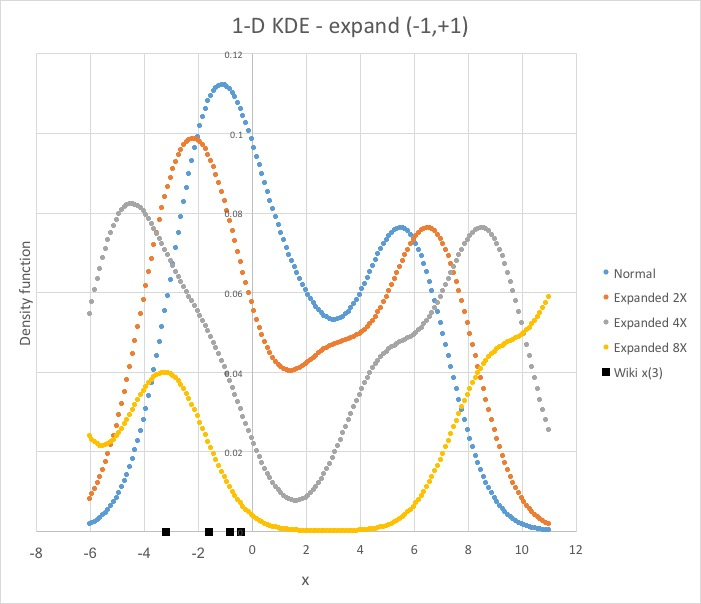There are several things to notice about the three expanded curves relative to the original:

• to the right of the origin, the main peak remains at the same height (0.076) because the three right-most kernels are fixed with respect to each other;
• a shoulder for x4 begins to emerge on the “expanded” curve as it separates from and loses the additive contribution from the kernel for x3;
• to the left of the origin, the height of the main peak drops as the central expansion pushes x1 and x2 away, effectively removing the kernel for x3 from the summation;
• a subtle shoulder due to the x3 kernel is visible on the orange (2X) curve, becoming more noticeable on the gray (4X) curve, finally appearing as a distinct peak in the yellow (8X) curve, as the effect of the first two kernels on x3 diminishes; and
• the valley between the two peaks (modes) in the original deepens and bottoms-out as the expansion removes any contribution from the near-zero kernel, or any of the others for that matter.

### The curse of empty space

This last bullet highlights — ironically by the absence of a “signal” where the yellow curve bottoms-out — that a univariate or bivariate kernel density estimate is usually a “local” construct. This situation changes dramatically as the number of dimensions goes up, for example, in machine-learning or other “big-data” situations. Here is an example (simplified and paraphrased from James et al., 2017, p. 108-9):

Imagine a data set with 100 points. In a univariate (1-D) analysis, that would be adequate to calculate an accurate KDE. If those same data were spread out over 20 dimensions, however, then any given observation would generally have no nearby neighbors, the so-called curse of dimensionality. A KDE can still be calculated, but now the bulk of the contributions will generally be from distant tails on the kernels, which plays havoc with the bias-variance trade-off (very roughly accuracy vs. precision) of the results.

The contrived example (above) illustrates conceptually how the curse of dimensionality, less commonly referred to as the “empty space phenomenon” (Silverman, 1986; Verleysen, 2003), might manifest in 1-D. No artifice is needed to see the equivalent in 2-D, however, because every time we use a log-ratio transformation on triad data we are unwittingly creating a scale expansion. Metaphorically, this allows a “leakage” of empty space into the near-vertex, near-zero data, stretching them apart so that the KDE is largely determined by the tails on no-longer-local kernels.

We can get a good feel for the potential magnitude of this effect by a simple coordinate-mapping exercise, going from a ternary to a rectilinear LRT plot. Here is a triad, sub-divided into two parts: the cleverly-named “interior” and a surrounding “annulus,” separated by the 1% coordinate lines for the respective vertices:In the triad coordinate frame, with a little help from Pythagoras, the base of the full triangle is 100 units; the height is 86.6 (= √3/2 x 100); and the area is 4330 units2 (= 1/2 x 100 x 86.6). The base of the interior triangle is 98 units; the height is 84.0 (= √3/2 x 97, not 98!); and the area is 4116. Hence the area of the annulus is 214 (= 4330 – 4116). The annulus/total ratio is 0.0494; and the annulus/interior is 0.0520. We will use the latter below for a comparison with the analogous ratios (notice the plural) in the LRT plot.

Additionally, we need some points in the triad for the coordinate-mapping exercise. Here is a representative cluster near the B vertex:### Two quick asides

A refresher: If you’ve never given much thought to how the shapes of data patterns can change between ternary and Cartesian plots — in this case between a triad and a log-ratio plot — the previous post in this series, Part VII: Mapping the Datasaurus Dozen, offers a visual primer of sorts. See especially the fourth and fifth figures down that page, the two adjacent multi-image panels. These are followed by a few paragraphs, under the “So what…?” heading, that summarize the workings of the log-ratio transformation.

A reminder: Now that we’re deep in the technical weeds, as I called them up in the main post, it’s worth recalling where we’re headed. What we’re after is a conceptual understanding of the mismatch between the location of story data in the triad T7 and the contours for their kernel density estimate. The hypothesis is that the log-ratio transformation, which is necessary to allow statistical analysis of the closed data from the triad, also introduces a distortion in near-vertex, near-zero data prior to the KDE calculation. We’re about to see how large that distortion, the stretching-out, can be.

### Some assembly required

Here are the near-vertex coordinate points for component B (above), mapped to a rectilinear grid with the isometric log-ratio (ilr) transformation (see Egozcue et al., 2003):The colored hexagons correspond to the respective locations in the triad — (0,100,0), (1,98,1), and (5,90,5) — and similarly for the 1% A and 1% C coordinate lines.  The curve for 95% B is a schematic fit, but close enough to hint that the overall pattern of coordinate lines is more complicated than in a triad. The solid arrows look like the BA and BC legs of the ternary, but they actually end at the midpoints of their respective legs (see below).

There are two important things to notice about the overall nature of this graph. Firstly, the annulus (see the second-previous figure, above) is now evident as the area bounded on the outside by the BA and BC legs emanating from the blue hexagon and on the inside by the two short dashed segments pointing away from the green hexagon to the upper-right and straight down. (The large rhomboid between the green and blue hexagons is part of the dog-leg shape of the annulus in this part of the graph.)

Secondly, the label on the blue hexagon, “~100% B,” indicates that it is only an approximation, as are the BA and BC legs, because logarithmic terms cannot have zero values. Instead, there are a variety of ad hoc methods for handling zeroes in LRTs (Aitchison, 1986, ch. 11; van den Boogaart and Tolosana-Delgado, 2013, ch. 7). The actual coordinates for the blue hexagon are (0.01,99.98,0.01) and similarly for the BA and BC legs. The point at the intersection of the BA leg and the 1% A line, for example, is (0.99,99.00,0.01). The choice of whether to compensate for c being 0.01 in the a vs. b coordinate is part of the ad hoc nature of handling zeroes.

Said differently, in principle, the blue hexagon is only one of an unlimited number of “~100% B” points. The RGB-hexagon altitude could be extended another “decade” to the upper left to (0.001,99.998,0.001); and then another decade after that to (0.0001,99.9998,0.0001); and on and on.

### The fully-assembled picture

We’re finally in a position to look at this ilr-transformation plot with the triad in its entirety (up to an arbitrary cut-off of the expansion). Here it is, with minimal labeling in the interest of reducing clutter: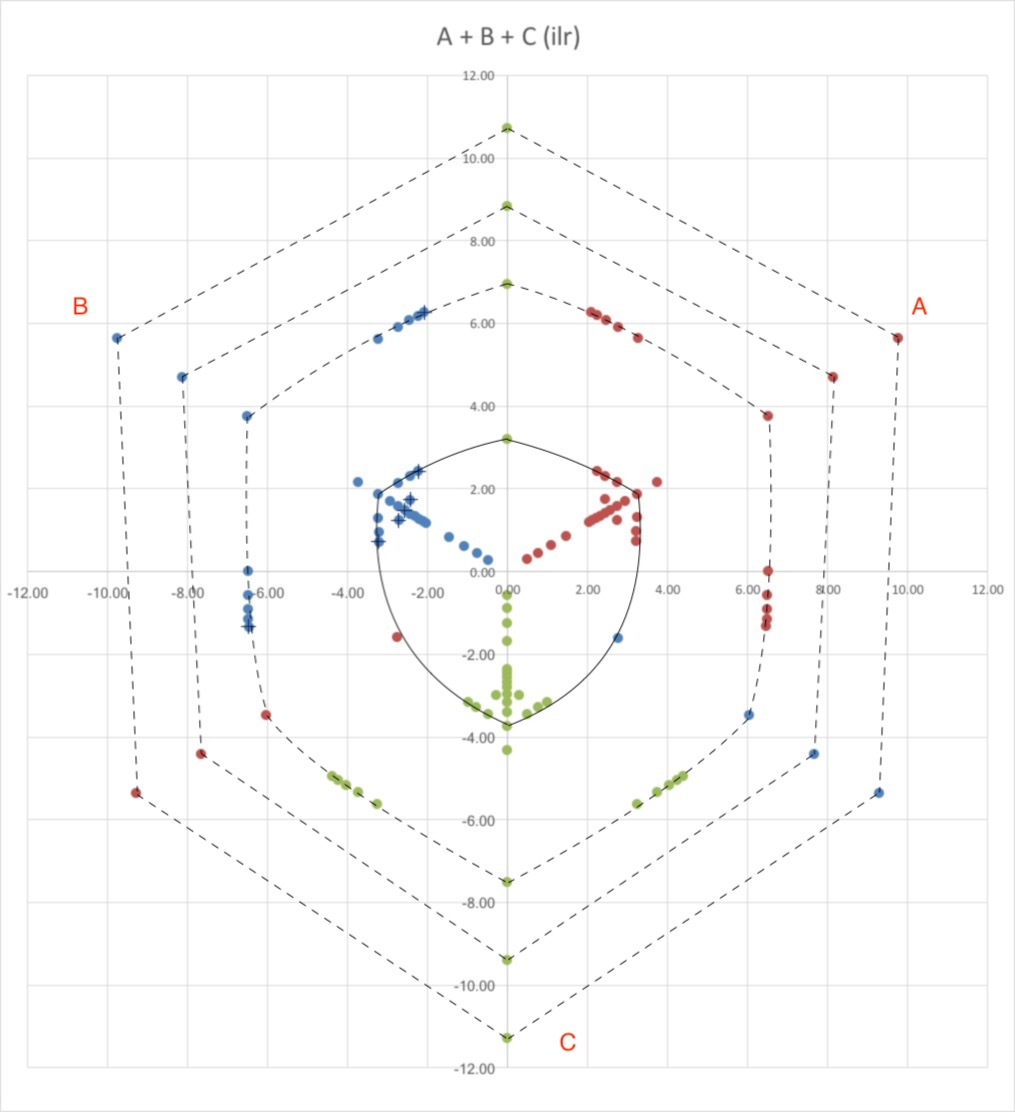• The color coding is A = red; B = blue; and C = green.
• The origin of the ilr plot (0,0) corresponds to the centroid in the ternary (33+,33+,33+).
• With a little study, the points and lines described in the previous figure for B should be identifiable for the other two vertices. The (+) signs mark the points connected by the 95%-B dashed line.
• The four solid line segments approximately locate the 1%-boundary between the interior and annulus in the triad. Recall that the enclosed interior is about 95% of the total area in standard ternary coordinates.
• The dashed line segments approximately locate the outside of the annular bands, with zeroes replaced successively by 0.01%, 0.001%, and 0.0001%. The straight-line approximation on the outer two bands underestimates the area of each annulus by a few percent.

I used the fabulous SketchAndCalc to measure the areas of each of the four enclosed shapes on a calibrated version of the image. The area of each annulus is then calculated by subtracting out the area of the interior teardrop; and the ratio of annulus/interior calculated for comparison with that ratio in a ternary. Recall that we found above that the latter was 0.0520. As the ilr plot clearly shows, each of the three annular shapes is larger than the interior. In fact, the annulus/interior ratios moving outward are 3.021, 5.462, and 8.440. And therefore the respective area “stretching” factor is very large: 58.1 (= 3.021/0.0520), 105, and 162.

Here are those “stretching” factors displayed as a function of the zero-replacement value for each annulus: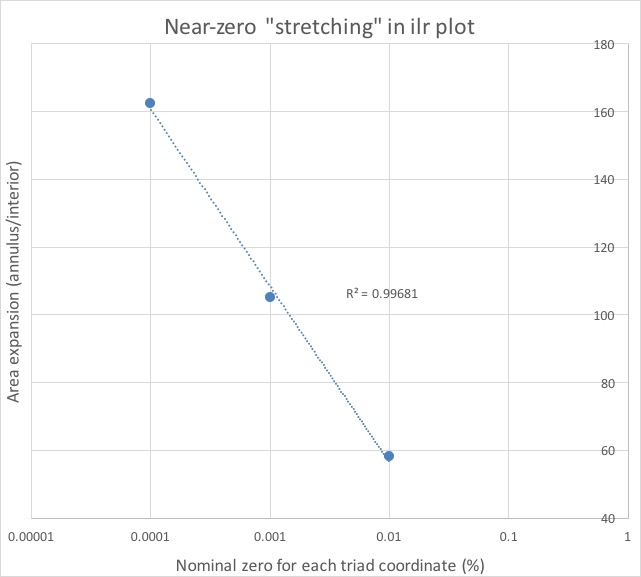Using more coordinate points for the outer annular bands in the ilr plot would improve on my straight-line approximation and perhaps improve the stretching-factor curve. Still, I’m happy with an R of almost three-nines.

Now we can connect the conceptual dots and see that the hypothesis we’ve been pursuing is not only plausible but compatible with both story collection and resulting analysis:

• Each of these decade expansions increases the area of the annulus as the BA and BC legs necessarily migrate out with it.
• Placement of signifier points by touching a screen or clicking a mouse or transcribing a pencil mark is relatively imprecise, but more importantly highly non-linear. Two story points that look “adjacent” to a casual observer may become “widely-separated” in the subsequent log-ratio transformation.
• Overall, the near-vertex, near-zero story points stretch out with the coordinate frame. (Conversely, though it wasn’t explicit above, near-centroid points will be squeezed together.)
• The usual fixed-width kernels get farther and farther apart in the annulus. When they are summed, the kernel density estimate worsens — the equivalent of the yellow (8X) curve bottoming-out in the histogram example (above).
• Thus the contours of the probability density function don’t play nicely with the story points.
• Q.E.D. Not QED Insight, just Q.E.D.

### So what (do we do to fix this)?

The solution is to use a variable kernel density estimation (footnotes added):

Using a fixed filter width may mean that in regions of low density, all samples will fall in the tails of the filter with very low weighting, while regions of high density will find an excessive number of samples in the central region with weighting close to unity. To fix this problem, we vary the width of the kernel in different regions of the sample space. There are two methods of doing this: balloon and pointwise estimation. In a balloon estimator, the kernel width is varied depending on the location of the test point.  In a pointwise estimator, the kernel width is varied depending on the location of the sample. 

These two estimators are also referred to as “locally-adaptive,” which shows their potential utility for the triad-to-LRT issue.  They are discussed in a number of sources, including Jones (1990) and Terrell and Scott (1992). Sain (2002) is particularly clear and has helpful illustrations from both real and simulated datasets. He also discusses the difficulties in actually implementing these estimators, including with respect to the bias-variance trade-off.

I suspect that these practical difficulties are the basis for two other things I have noticed in learning about KDE and especially variable/locally-adaptive methods. Firstly, it is not easy to find more recent literature on the development and extension of the subject, as opposed to applications.  I have not looked at Scott (2015), the second edition of one of the three major monographs, so I may have simply missed the latest sources. Nonetheless, it is a bit odd that Terrell and Scott (1992) from 25 years ago is the most recent methodological citation in the Wikipedia article (link above).

Secondly, in the world of R, it appears that kde2d, the KDE function from the MASS package, does not include any variable-kernel capability. Hence, ggtern, the package of choice for producing triads in SenseMaker projects, cannot invoke it (although I am awaiting confirmation of this from Nicholas Hamilton). In fact, a Google-based search of the r-project.org site reveals only a few packages (such as ‘kader’, ‘np’, and ‘sparr’) that include any kind of multivariate adaptive-estimator or variable-bandwidth capability. Based on my reading of the descriptions, it is not obvious that they are a useful resource.

Instead, at least for the near term, ternary heat maps are now available in ggtern, brought to you by the aforementioned Nicholas Hamilton (and partly supported by QEDInsight), with your choice of triangular or hexagonal bins. Some SenseMaker examples are shown in Part VIIIb.

### A final note

Non-SenseMaker users of ternary graphs who routinely calculate KDE-PDF contours, whether with an R package such as ggtern or with home-grown code, could be forgiven for asking why no one else has remarked on the problem discussed in this post. Great question. Why indeed? Here are three possibilities:

Firstly, people are just plotting the contours and assuming they’re right, with little to no comparison back to the original data. While my cynical side might be happy with that explanation, I also know that most people who spend the time and effort required to produce these graphs are very invested in them. More to the point, we now know of at least seven people working with story data who have noticed the mismatch in multiple projects. No offense to these people (or ourselves), but SenseMaker practitioners have not cornered the market on careful inspection of data. Almost everyone does that, so the answer must lie somewhere else.

Secondly, three members of the group of Spanish mathematicians that has extended Aitchison’s original work on compositional analysis over the past 20 years have looked at kernel density estimates in ternary and rectilinear diagrams. Martín-Fernández et al. (2007) used a dataset of basaltic lavas from Aitchison (1986), which shows the type of centered, arcuate pattern that we first encountered here in Part II: Log-Ratio Transformation. Subsequently, they did extensive simulations with a group of 12 test densities to compare several kernel estimators, using standard mean integrated square error measures.

Here are the 12 test densities from Chacón (2009) in rectilinear coordinates: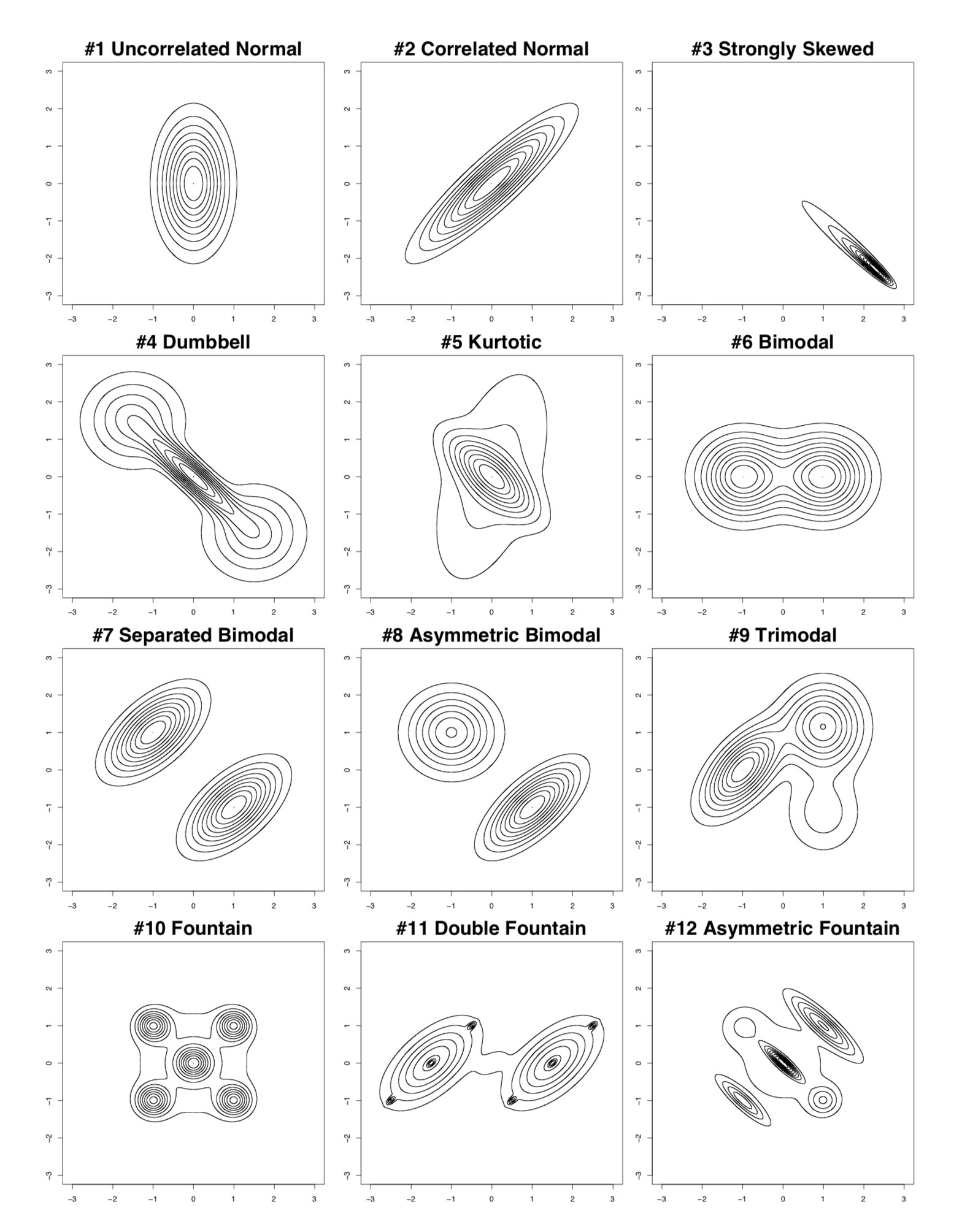And here are the same 12 test densities from Chacón et al. (2008) in ternary coordinates (inverse-transformed from the square grid):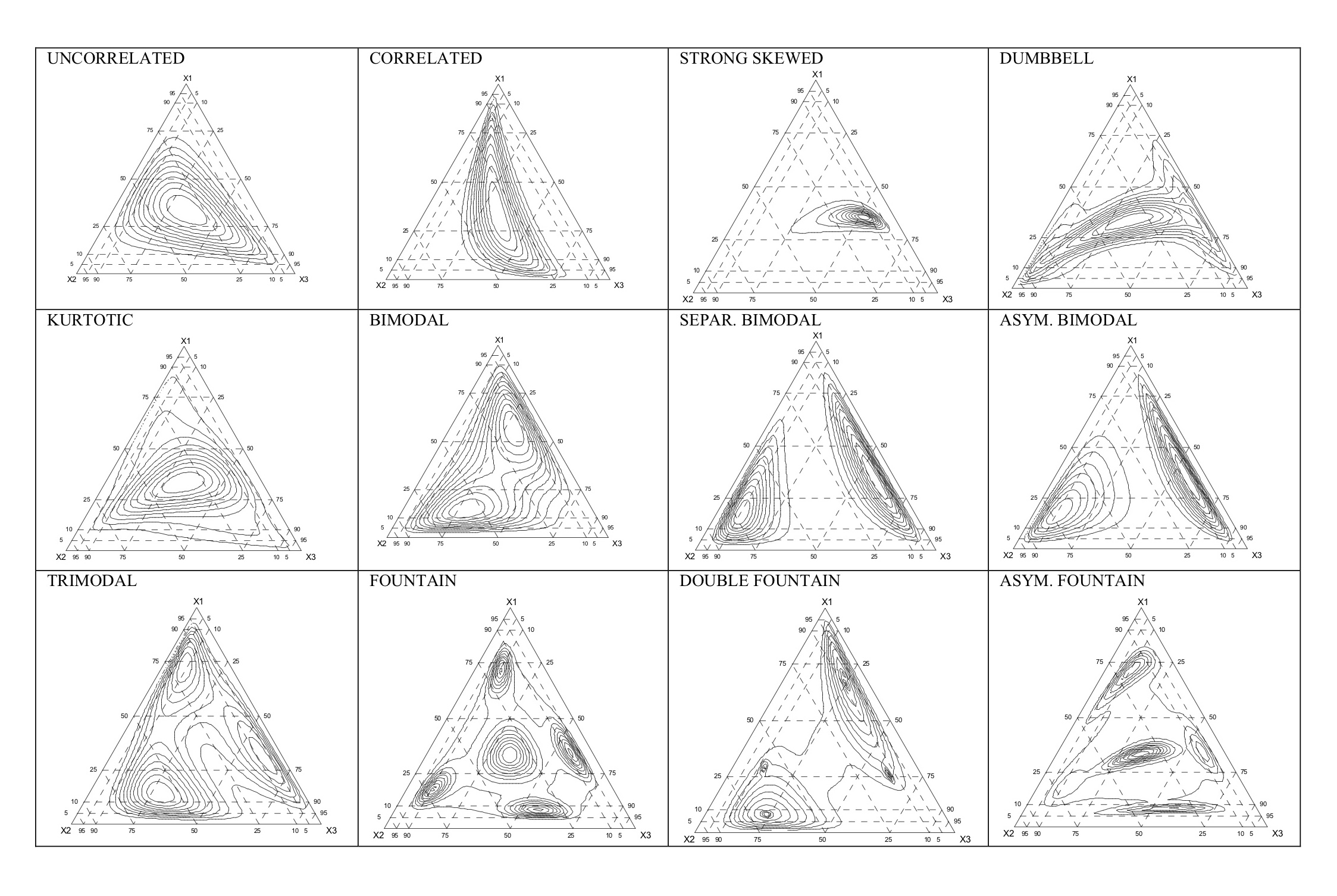These two sets of contours are not explicitly from the same sample set for each density, but Chacón et al. (2008) described them as “closely related.” The methodology is certainly close enough for the purposes of this post.

Note in particular that all 12 densities in the grid plots are (1) centered on the origin, which we saw above corresponds to the centroid of the triad; and (2) fall within, generally well within, the range of (-3,+3) on each of the two axes. Compare this to the range of (-12,+12) in the “stretched” ilr-transformed figure above. As constructed, these simulated patterns would fall within the solid-line-bounded “teardrop” in our stretched plot. Thus, it is no surprise that the contours for the 12 test densities almost completely miss the “0-1%” annulus in the ternary plots. Said differently, if you set out to avoid having a near-vertex, near-zero stretching effect in ternary data, it’s hard to imagine a better demonstration of how and why it could go unnoticed.

Thirdly, there is the flip side of the previous point. A triad in a SenseMaker project is not only a data display tool, but it is also a data collection tool. Efforts to balance the signifiers at all three vertices and to avoid influencing respondents notwithstanding, every project and triad has finger touches and cursor clicks on edges and corners. By design, storytellers are allowed to choose near-vertex, near-zero placements, in some sense encouraged to do so by their view of the labels. If you think about the larger universe of data presentation in ternary diagrams, this is an unusual situation, one very unlikely to be encountered, I suspect, by a metallurgist or geologist or ecologist or geneticist.

### References

Aitchison, J. (1986, reprinted 2003) The Statistical Analysis of Compositional Data. The Blackburn Press, Caldwell NJ. 416 pp. plus additional material.

Chacón, J.E. (2009) Data-driven choice of the smoothing parametrization for kernel density estimators. The Canadian Journal of Statistics, v. 37, p. 249-265.

Chacón, J.E., Martín-Fernández, J.A., and Mateu-Figueras, G. (2008) A comparison of the alr and ilr transformation for kernel density estimation of compositional data. CoDaWork’08, 3rd Compositional Data Analysis Workshop, Girona (Spain), May 2008. Retrieved February 2018 from https://dugi-doc.udg.edu/bitstream/handle/10256/724/Chacon.pdf

Duong, T., and Hazelton, M.L. (2003) Plug-in bandwidth matrices for bivariate kernel density estimation. Nonparametric Statistics, v. 15, p. 17-30.

Duong, T., and Hazelton, M.L. (2005) Cross-validation bandwidth matrices for multivariate kernel density estimation. Scandinavian Journal of Statistics, v. 32, p. 485-506.

Egozcue, J.J., Pawlowsky-Glahn, V., Mateu-Figueras, G., and Barceló-Vidal, C. (2003) Isometric logratio transformation for compositional data analysis. Mathematical Geology, v. 35, p. 279-300.

Hastie, T., Tibshirani, R., and Friedman, J. (2016, corrected 12th printing 2017) The Elements of Statistical Learning, second edition. Springer, New York. 745 pp.

James, G., Witten, D., Hastie, T., and Tibshirani, R. (2013, corrected 8th printing 2017) An Introduction to Statistical Learning with Applications in R. Springer, New York. 440 pp.

Jones, M.C. (1990) Variable kernel density estimates and variable kernel density estimates [sic]. Australian Journal of Statistics, v. 32, p. 361-371.

Martín-Fernández, J.A., Chacón-Durán, J.E., and Mateu-Figueras, G. (2007) Updating on the kernel density estimation for compositional data, pp. 713-720 in Rizzi, A., and Vichi, M. eds., COMPSTAT 2006 – Proceedings in Computational Statistics, Rome. Springer Physica-Verlag, Heidelberg.

Sain, S.R. (2002) Multivariate locally adaptive density estimation. Computational Statistics & Data Analysis, v. 39, p. 165-186.

Scott, D.W. (2015) Multivariate Density Estimation, second edition. Wiley, Hoboken NJ. 384 pp.

Shimazaki, H. and Shinomoto, S. (2010) Kernel bandwidth optimization in spike rate estimation. Journal of Computational Neuroscience, v. 29, p. 171-182.

Silverman, B.W. (1986, reprinted 1998) Density Estimation for Statistics and Data Analysis. Chapman & Hall/CRC, Boca Raton. 175 pp.

Terrell, G.R., and Scott, D.W. (1992) Variable kernel density estimation. The annals of Statistics, v. 20, p. 1236-1265.

van den Boogaart, K.G., and Tolosana-Delgado, R. (2013) Analyzing Compositional Data with R. Springer-Verlag, Berlin. 258 pp.

Verleysen, M. (2003) Learning high-dimensional data, pp. 141-162 in Ablameyko, S., et al., eds., Limitations and Future Trends in Neural Computation, NATO Science Series, III: Computer and Systems Sciences, v. 186. IOS Press, Amsterdam.

### Footnotes

1. By Drleft at English Wikipedia, CC BY-SA 3.0, Link  ^
2. This description says only that “the kernels are summed,” but the formal definition is that the estimated density function f(xo) at target point xo is the average of the n kernels contributing at that location. It is common practice, however, to plot nf(xo) in the interest of clarity (for example, Jones, 1990, and Hastie et al., 2009), and I have done that here.  ^
3. In Annie Hall, Woody Allen’s childhood surrogate, Little Alvy, is suffering from existential angst upon learning that the universe is expanding. He concludes that life is pointless and, perhaps a bit disingenuously, stops doing his homework. To which his mother responds: “What has the universe got to do with it? You’re here in Brooklyn. Brooklyn is not expanding!”  ^
4. These two lines are correctly labelled. Even though A and C are at the lower-left and lower-right vertices, respectively, of the triad, the LRT reverses them, and simultaneously rotates the triad roughly 60° CCW. This is well-known behavior for the LRTs, which is easier to see with the alr transformations in Part VII: Mapping the Datasaurus Dozen.  ^
5. A test point is also known as an estimation point or query point or target point. It is a location on the number line or computational grid where the sum/average of the PDF is calculated. All those colored dots in the 2X/4X/8X expanded curves are calculated at target points.  ^
6. A sample location is also known as a data point or an observation. The six kernels (red dashed curves) in the univariate histogram-KDE comparative plot are centered on the six sample locations.  ^
7. Martín-Fernández et al. (2007) illustrate the use of bivariate kernel estimators from Duong and Hazelton (2003, 2005) with compositional data in ternaries. These plug-in and cross-validation methods are full-bandwidth matrix extensions that can accommodate not only axis-parallel and diagonal datasets, but more general and multi-modal distributions. They are data-driven and may start from pilot bandwidths, but they are not locally-adaptive and thus do not address the triad-to-LRT stretching issue.  ^
8. But see Shimazaki and Shinomoto (2010), which is both developmental and applied. This paper looks at timeline-based neurological spikes. The conceptual parallel is so strong, however, that it is hard to believe their method for selecting bandwidth “locally in time, assuming a non-stationary rate modulation” can’t be ported over to more conventional KDE analyses.  ^